Скачать презентацию UNIVERSITY OF MASSACHUSETTS Dept of Electrical Computer

75d25616b9e8c622543bf2030aecbc3d.ppt

• Количество слайдов: 24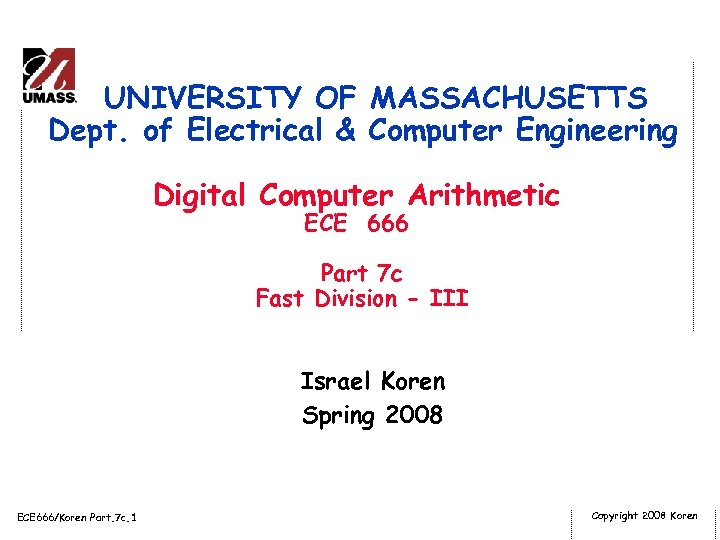UNIVERSITY OF MASSACHUSETTS Dept. of Electrical & Computer Engineering Digital Computer Arithmetic ECE 666 Part 7 c Fast Division - III Israel Koren Spring 2008 ECE 666/Koren Part. 7 c. 1 Copyright 2008 Koren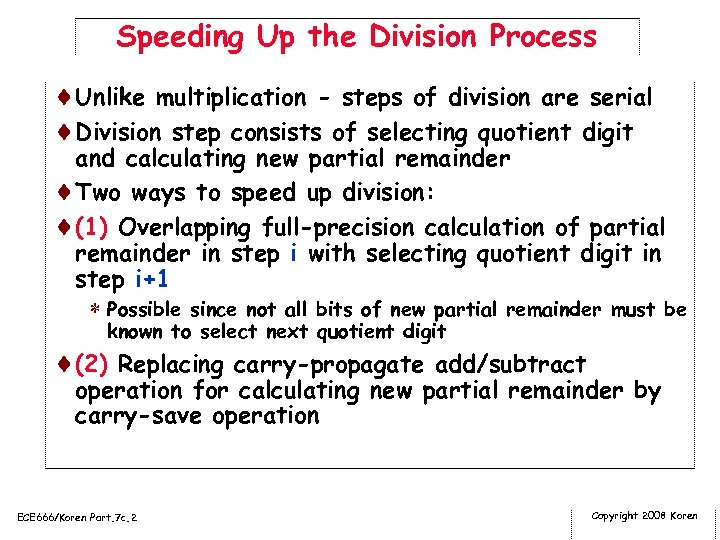Speeding Up the Division Process ¨Unlike multiplication - steps of division are serial ¨Division step consists of selecting quotient digit and calculating new partial remainder ¨Two ways to speed up division: ¨(1) Overlapping full-precision calculation of partial remainder in step i with selecting quotient digit in step i+1 * Possible since not all bits of new partial remainder must be known to select next quotient digit ¨(2) Replacing carry-propagate add/subtract operation for calculating new partial remainder by carry-save operation ECE 666/Koren Part. 7 c. 2 Copyright 2008 Koren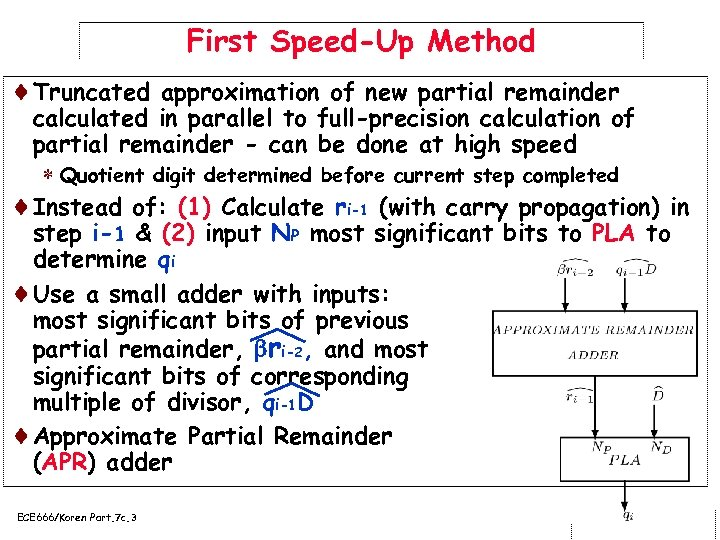First Speed-Up Method ¨Truncated approximation of new partial remainder calculated in parallel to full-precision calculation of partial remainder - can be done at high speed * Quotient digit determined before current step completed ¨Instead of: (1) Calculate ri-1 (with carry propagation) in step i-1 & (2) input NP most significant bits to PLA to determine qi ¨Use a small adder with inputs: most significant bits of previous partial remainder, ri-2, and most significant bits of corresponding multiple of divisor, qi-1 D ¨Approximate Partial Remainder (APR) adder ECE 666/Koren Part. 7 c. 3 Copyright 2008 Koren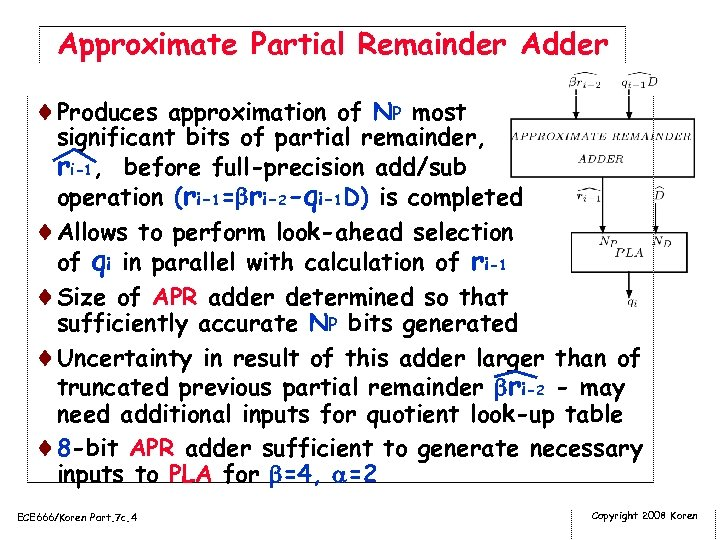Approximate Partial Remainder Adder ¨Produces approximation of NP most significant bits of partial remainder, ri-1, before full-precision add/sub operation (ri-1= ri-2 -qi-1 D) is completed ¨Allows to perform look-ahead selection of qi in parallel with calculation of ri-1 ¨Size of APR adder determined so that sufficiently accurate NP bits generated ¨Uncertainty in result of this adder larger than of truncated previous partial remainder ri-2 - may need additional inputs for quotient look-up table ¨ 8 -bit APR adder sufficient to generate necessary inputs to PLA for =4, =2 ECE 666/Koren Part. 7 c. 4 Copyright 2008 Koren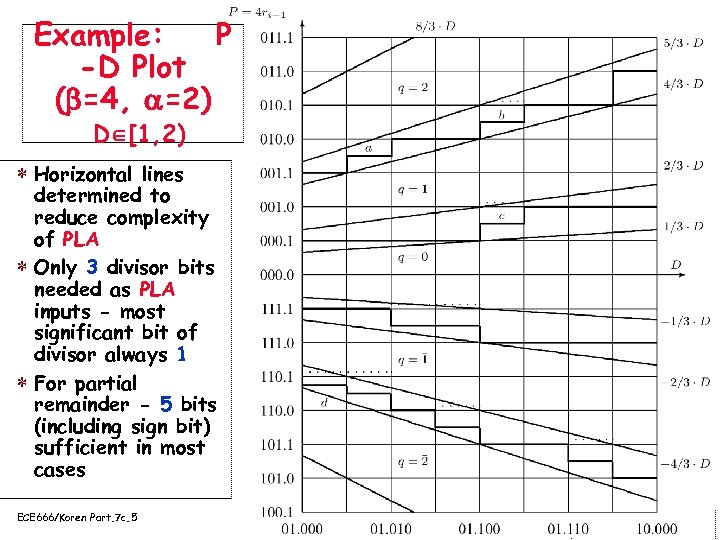Example: P -D Plot ( =4, =2) D [1, 2) * Horizontal lines determined to reduce complexity of PLA * Only 3 divisor bits needed as PLA inputs - most significant bit of divisor always 1 * For partial remainder - 5 bits (including sign bit) sufficient in most cases ECE 666/Koren Part. 7 c. 5 Copyright 2008 Koren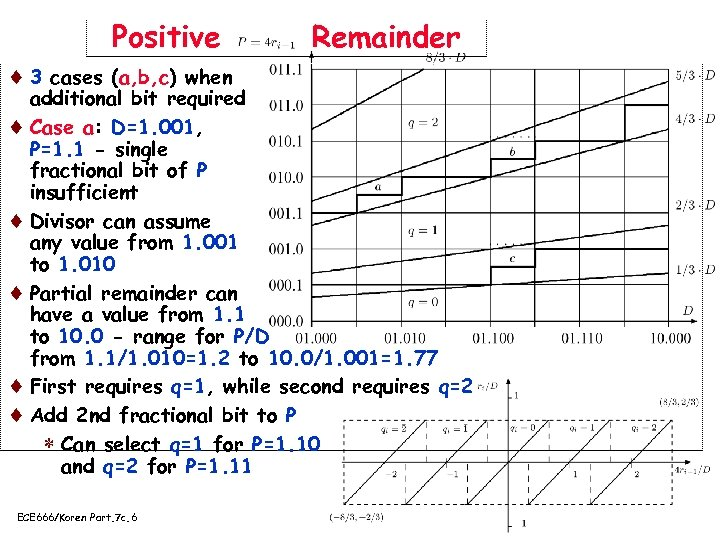Positive Remainder ¨ 3 cases (a, b, c) when additional bit required ¨ Case a: D=1. 001, P=1. 1 - single fractional bit of P insufficient ¨ Divisor can assume any value from 1. 001 to 1. 010 ¨ Partial remainder can have a value from 1. 1 to 10. 0 - range for P/D from 1. 1/1. 010=1. 2 to 10. 0/1. 001=1. 77 ¨ First requires q=1, while second requires q=2 ¨ Add 2 nd fractional bit to P * Can select q=1 for P=1. 10 and q=2 for P=1. 11 ECE 666/Koren Part. 7 c. 6 Copyright 2008 Koren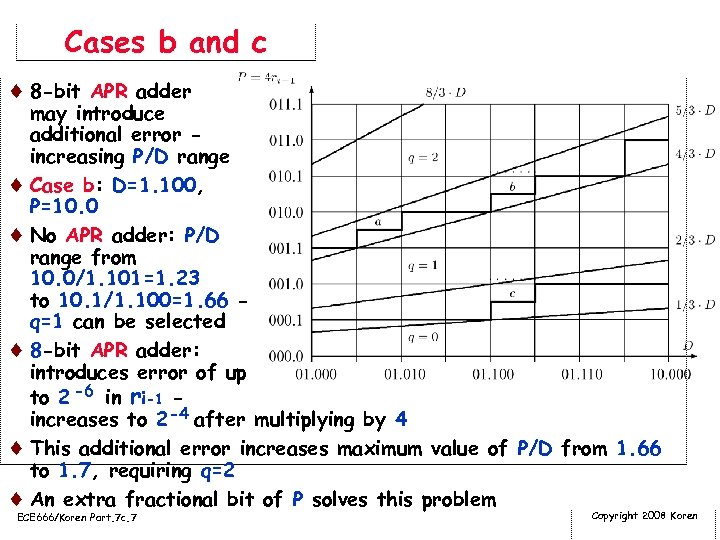Cases b and c ¨ 8 -bit APR adder may introduce additional error increasing P/D range ¨ Case b: D=1. 100, P=10. 0 ¨ No APR adder: P/D range from 10. 0/1. 101=1. 23 to 10. 1/1. 100=1. 66 q=1 can be selected ¨ 8 -bit APR adder: introduces error of up to 2 -6 in ri-1 increases to 2 -4 after multiplying by 4 ¨ This additional error increases maximum value of P/D from 1. 66 to 1. 7, requiring q=2 ¨ An extra fractional bit of P solves this problem ECE 666/Koren Part. 7 c. 7 Copyright 2008 Koren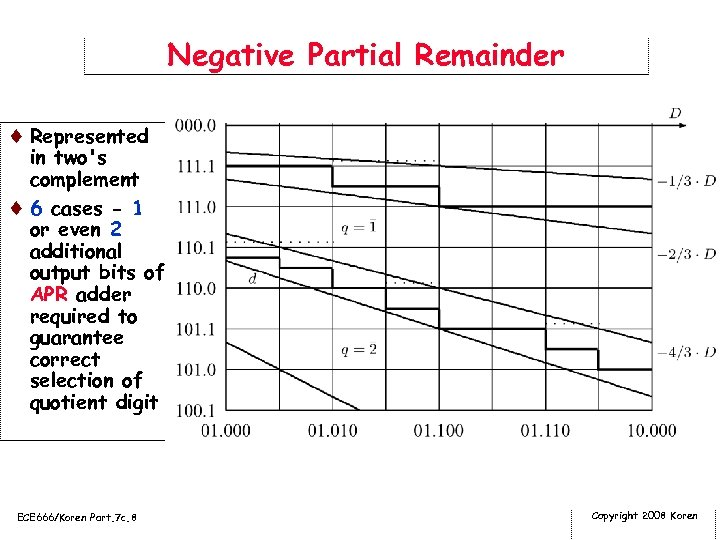Negative Partial Remainder ¨ Represented in two's complement ¨ 6 cases - 1 or even 2 additional output bits of APR adder required to guarantee correct selection of quotient digit ECE 666/Koren Part. 7 c. 8 Copyright 2008 Koren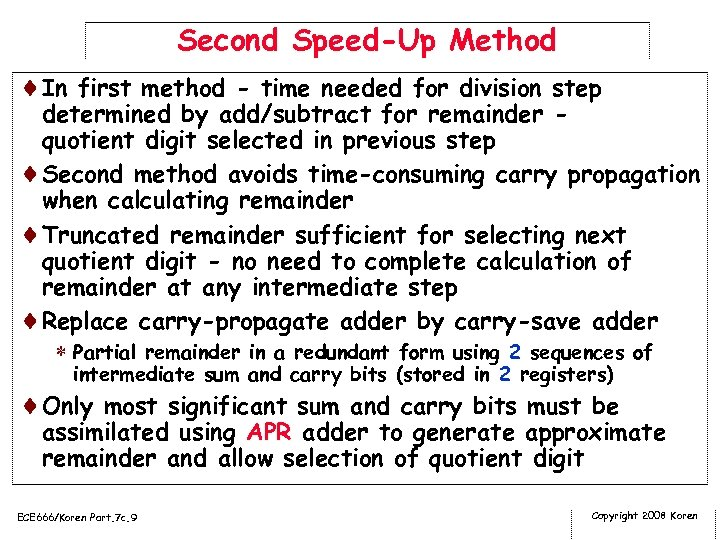Second Speed-Up Method ¨In first method - time needed for division step determined by add/subtract for remainder quotient digit selected in previous step ¨Second method avoids time-consuming carry propagation when calculating remainder ¨Truncated remainder sufficient for selecting next quotient digit - no need to complete calculation of remainder at any intermediate step ¨Replace carry-propagate adder by carry-save adder * Partial remainder in a redundant form using 2 sequences of intermediate sum and carry bits (stored in 2 registers) ¨Only most significant sum and carry bits must be assimilated using APR adder to generate approximate remainder and allow selection of quotient digit ECE 666/Koren Part. 7 c. 9 Copyright 2008 Koren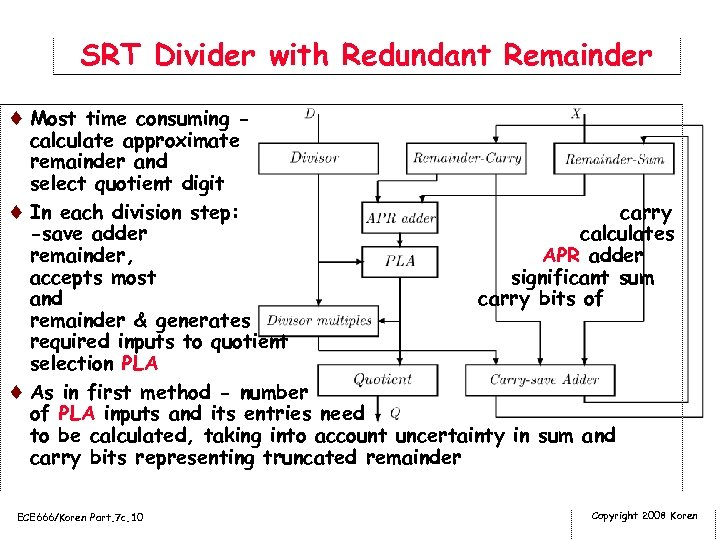SRT Divider with Redundant Remainder ¨ Most time consuming - calculate approximate remainder and select quotient digit ¨ In each division step: carry -save adder calculates remainder, APR adder accepts most significant sum and carry bits of remainder & generates required inputs to quotient selection PLA ¨ As in first method - number of PLA inputs and its entries need to be calculated, taking into account uncertainty in sum and carry bits representing truncated remainder ECE 666/Koren Part. 7 c. 10 Copyright 2008 Koren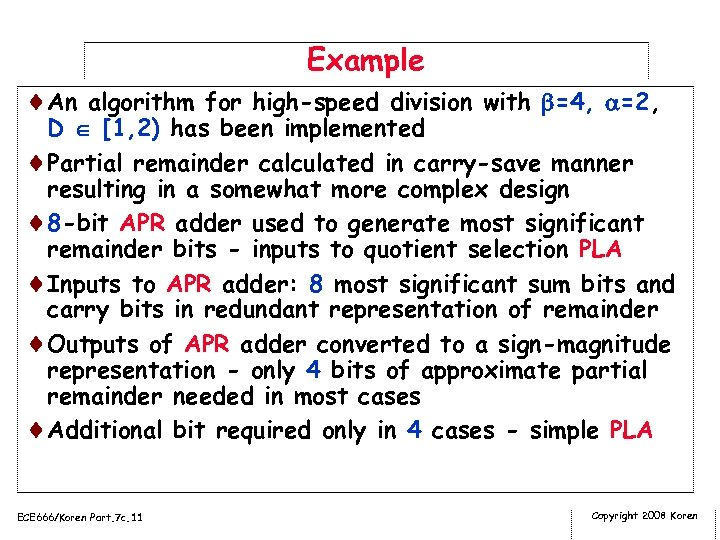Example ¨An algorithm for high-speed division with =4, =2, D [1, 2) has been implemented ¨Partial remainder calculated in carry-save manner resulting in a somewhat more complex design ¨ 8 -bit APR adder used to generate most significant remainder bits - inputs to quotient selection PLA ¨Inputs to APR adder: 8 most significant sum bits and carry bits in redundant representation of remainder ¨Outputs of APR adder converted to a sign-magnitude representation - only 4 bits of approximate partial remainder needed in most cases ¨Additional bit required only in 4 cases - simple PLA ECE 666/Koren Part. 7 c. 11 Copyright 2008 Koren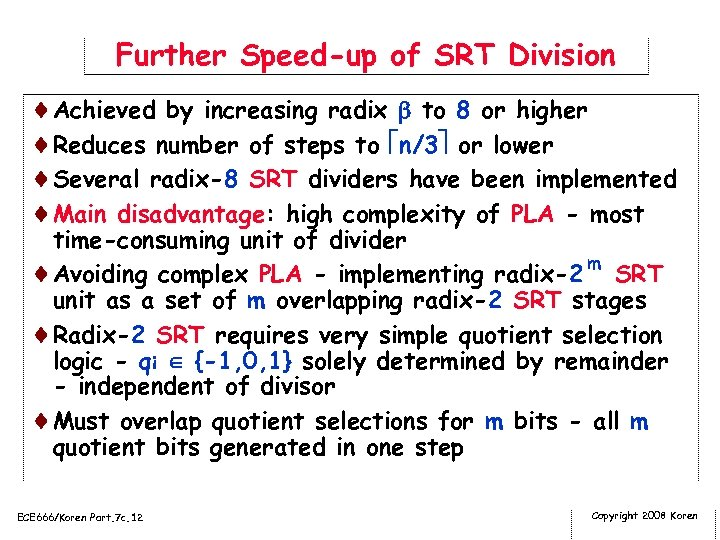Further Speed-up of SRT Division ¨Achieved by increasing radix to 8 or higher ¨Reduces number of steps to n/3 or lower ¨Several radix-8 SRT dividers have been implemented ¨Main disadvantage: high complexity of PLA - most time-consuming unit of divider ¨Avoiding complex PLA - implementing radix-2 m SRT unit as a set of m overlapping radix-2 SRT stages ¨Radix-2 SRT requires very simple quotient selection logic - qi {-1, 0, 1} solely determined by remainder - independent of divisor ¨Must overlap quotient selections for m bits - all m quotient bits generated in one step ECE 666/Koren Part. 7 c. 12 Copyright 2008 Koren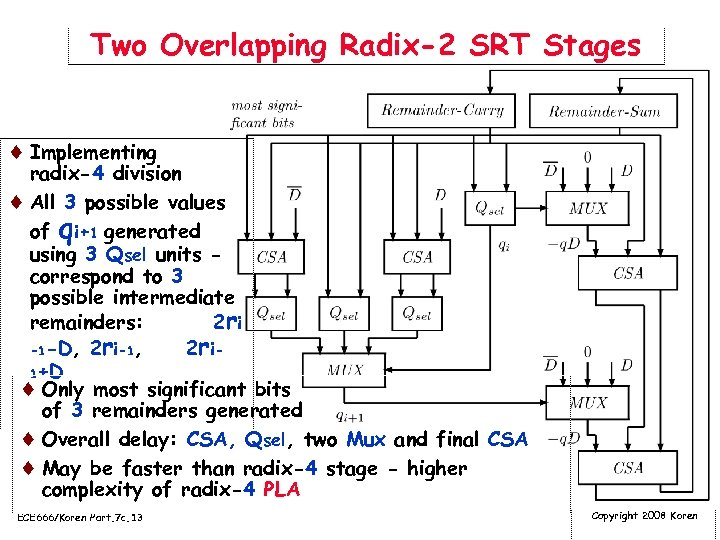Two Overlapping Radix-2 SRT Stages ¨ Implementing radix-4 division ¨ All 3 possible values of qi+1 generated using 3 Qsel units correspond to 3 possible intermediate remainders: 2 r i -1 -D, 2 ri-1, 2 r i 1+D ¨ Only most significant bits of 3 remainders generated ¨ Overall delay: CSA, Qsel, two Mux and final CSA ¨ May be faster than radix-4 stage - higher complexity of radix-4 PLA ECE 666/Koren Part. 7 c. 13 Copyright 2008 Koren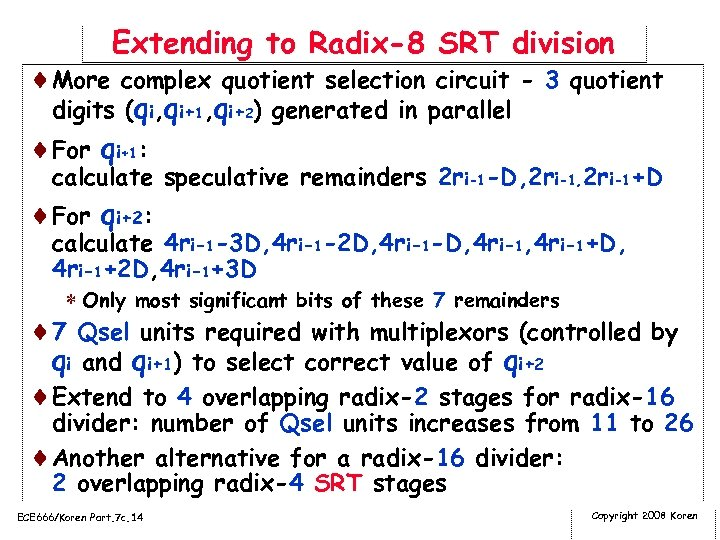Extending to Radix-8 SRT division ¨More complex quotient selection circuit - 3 quotient digits (qi, qi+1, qi+2) generated in parallel ¨For qi+1: calculate speculative remainders 2 ri-1 -D, 2 ri-1+D ¨For qi+2: calculate 4 ri-1 -3 D, 4 ri-1 -2 D, 4 ri-1 -D, 4 ri-1+D, 4 ri-1+2 D, 4 ri-1+3 D * Only most significant bits of these 7 remainders ¨ 7 Qsel units required with multiplexors (controlled by qi and qi+1) to select correct value of qi+2 ¨Extend to 4 overlapping radix-2 stages for radix-16 divider: number of Qsel units increases from 11 to 26 ¨Another alternative for a radix-16 divider: 2 overlapping radix-4 SRT stages ECE 666/Koren Part. 7 c. 14 Copyright 2008 Koren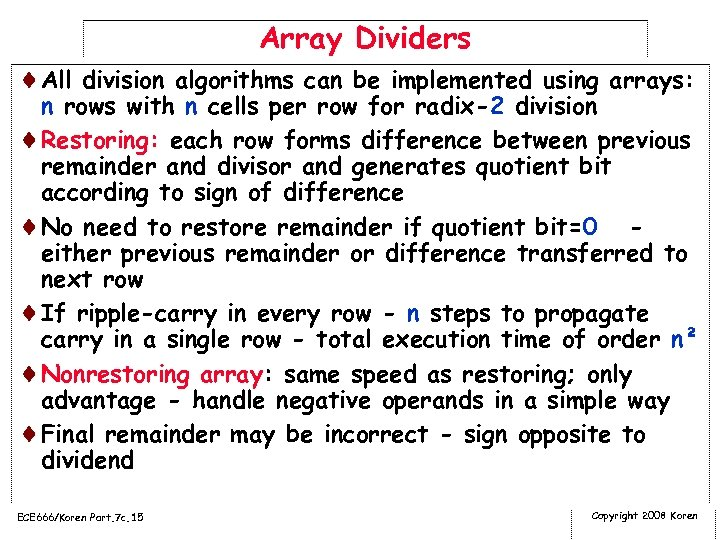Array Dividers ¨All division algorithms can be implemented using arrays: n rows with n cells per row for radix-2 division ¨Restoring: each row forms difference between previous remainder and divisor and generates quotient bit according to sign of difference ¨No need to restore remainder if quotient bit=0 either previous remainder or difference transferred to next row ¨If ripple-carry in every row - n steps to propagate carry in a single row - total execution time of order n² ¨Nonrestoring array: same speed as restoring; only advantage - handle negative operands in a simple way ¨Final remainder may be incorrect - sign opposite to dividend ECE 666/Koren Part. 7 c. 15 Copyright 2008 Koren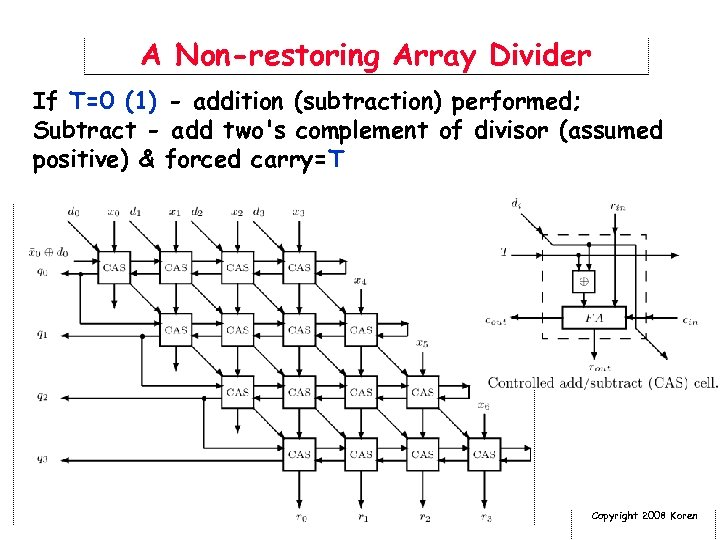A Non-restoring Array Divider If T=0 (1) - addition (subtraction) performed; Subtract - add two's complement of divisor (assumed positive) & forced carry=T ECE 666/Koren Part. 7 c. 16 Copyright 2008 Koren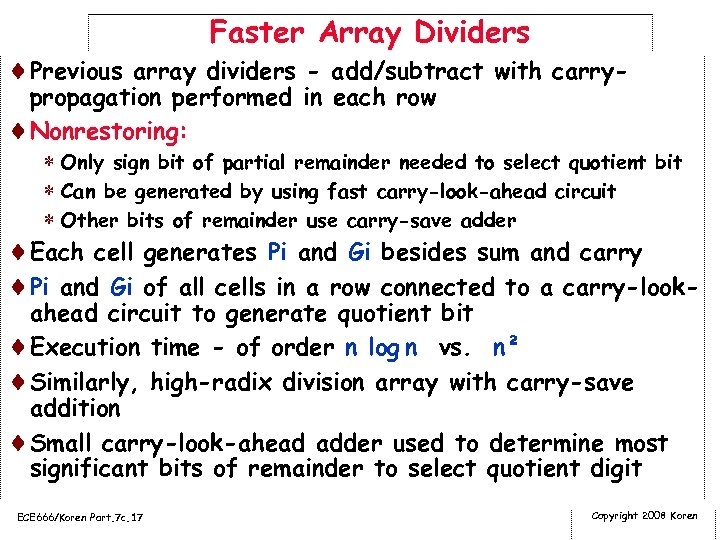Faster Array Dividers ¨Previous array dividers - add/subtract with carry- propagation performed in each row ¨Nonrestoring: * Only sign bit of partial remainder needed to select quotient bit * Can be generated by using fast carry-look-ahead circuit * Other bits of remainder use carry-save adder ¨Each cell generates Pi and Gi besides sum and carry ¨Pi and Gi of all cells in a row connected to a carry-lookahead circuit to generate quotient bit ¨Execution time - of order n log n vs. n² ¨Similarly, high-radix division array with carry-save addition ¨Small carry-look-ahead adder used to determine most significant bits of remainder to select quotient digit ECE 666/Koren Part. 7 c. 17 Copyright 2008 Koren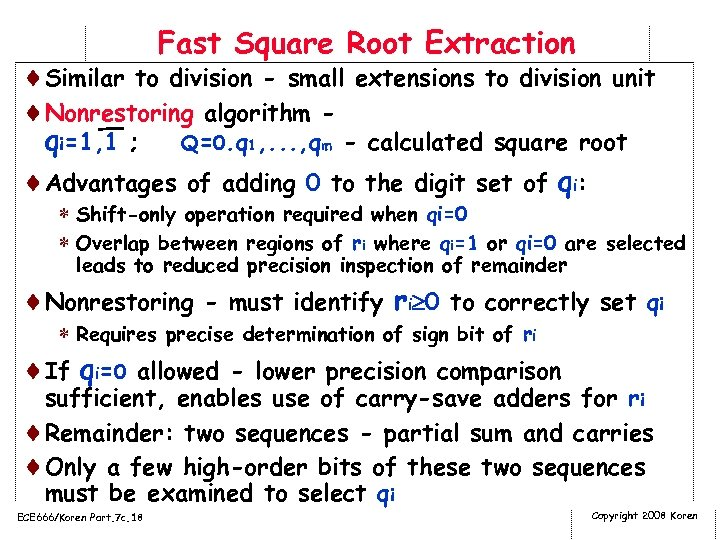Fast Square Root Extraction ¨Similar to division - small extensions to division unit ¨Nonrestoring algorithm qi=1, 1 ; Q=0. q 1, . . . , qm - calculated square root ¨Advantages of adding 0 to the digit set of qi: * Shift-only operation required when qi=0 * Overlap between regions of ri where qi=1 or qi=0 are selected leads to reduced precision inspection of remainder ¨Nonrestoring - must identify ri 0 to correctly set qi * Requires precise determination of sign bit of ri ¨If qi=0 allowed - lower precision comparison sufficient, enables use of carry-save adders for ri ¨Remainder: two sequences - partial sum and carries ¨Only a few high-order bits of these two sequences must be examined to select qi ECE 666/Koren Part. 7 c. 18 Copyright 2008 Koren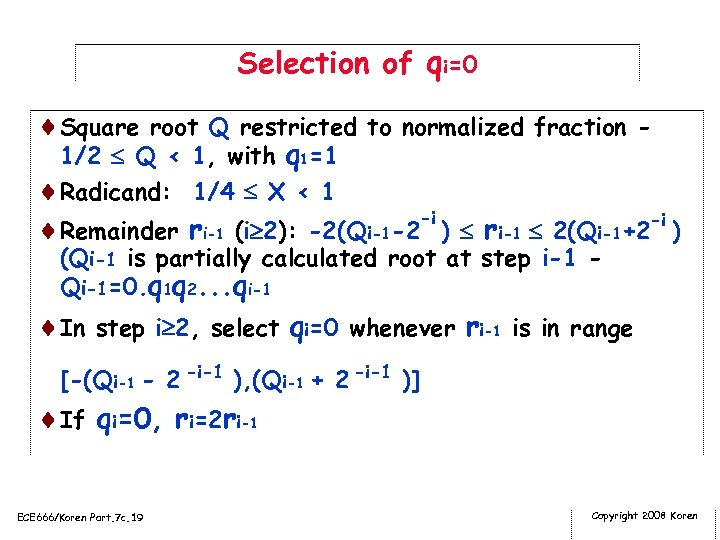Selection of qi=0 ¨Square root Q restricted to normalized fraction 1/2 Q < 1, with q 1=1 ¨Radicand: 1/4 X < 1 -i ¨Remainder ri-1 (i 2): -2(Qi-1 -2 ) ri-1 2(Qi-1+2 -i ) (Qi-1 is partially calculated root at step i-1 Qi-1=0. q 1 q 2. . . qi-1 ¨In step i 2, select qi=0 whenever ri-1 is in range [-(Qi-1 - 2 -i-1 ), (Qi-1 + 2 -i-1 )] ¨If qi=0, ri=2 ri-1 ECE 666/Koren Part. 7 c. 19 Copyright 2008 Koren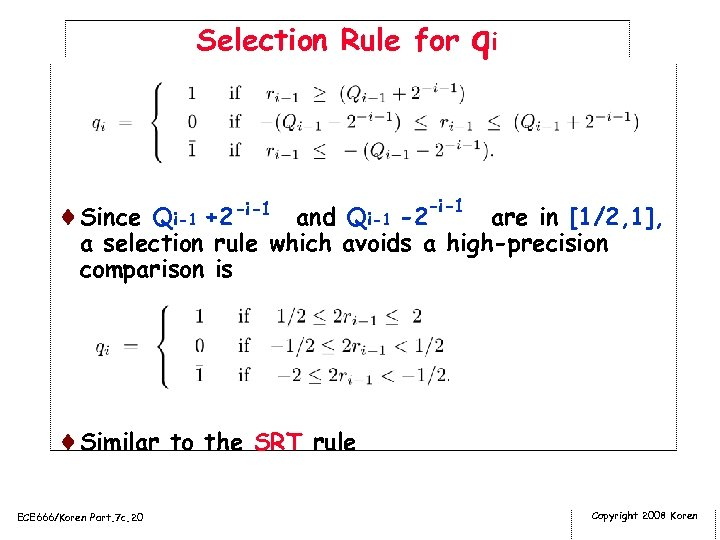Selection Rule for qi ¨Since Qi-1 +2 -i-1 and Qi-1 -2 -i-1 are in [1/2, 1], a selection rule which avoids a high-precision comparison is ¨Similar to the SRT rule ECE 666/Koren Part. 7 c. 20 Copyright 2008 Koren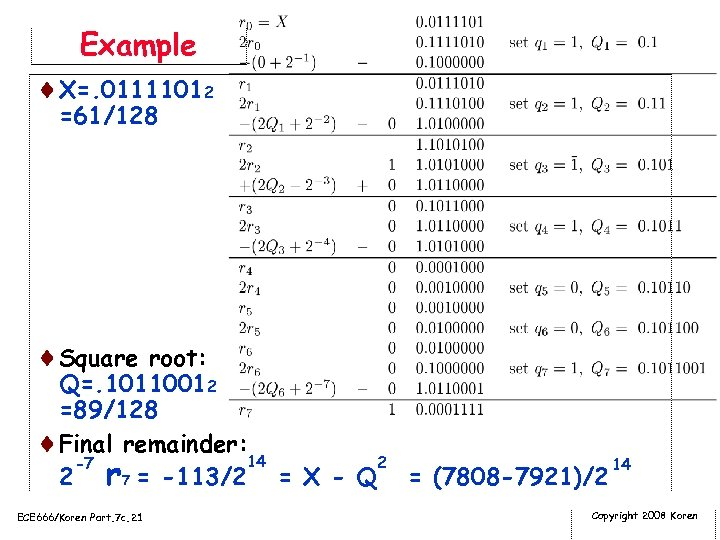Example ¨X=. 01111012 =61/128 ¨Square root: Q=. 10110012 =89/128 ¨Final remainder: 14 2 -7 14 2 r 7 = -113/2 = X - Q = (7808 -7921)/2 ECE 666/Koren Part. 7 c. 21 Copyright 2008 Koren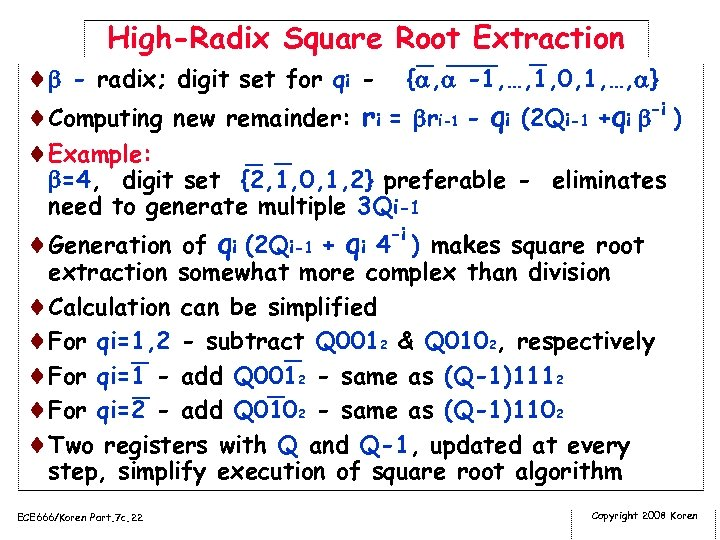High-Radix Square Root Extraction ¨ - radix; digit set for qi - { , -1, …, 1, 0, 1, …, } ¨Computing new remainder: ri = ri-1 - qi (2 Qi-1 +qi -i ) ¨Example: =4, digit set {2, 1, 0, 1, 2} preferable - eliminates need to generate multiple 3 Qi-1 -i ¨Generation of qi (2 Qi-1 + qi 4 ) makes square root extraction somewhat more complex than division ¨Calculation can be simplified ¨For qi=1, 2 - subtract Q 0012 & Q 0102, respectively ¨For qi=1 - add Q 0012 - same as (Q-1)1112 ¨For qi=2 - add Q 0102 - same as (Q-1)1102 ¨Two registers with Q and Q-1, updated at every step, simplify execution of square root algorithm ECE 666/Koren Part. 7 c. 22 Copyright 2008 Koren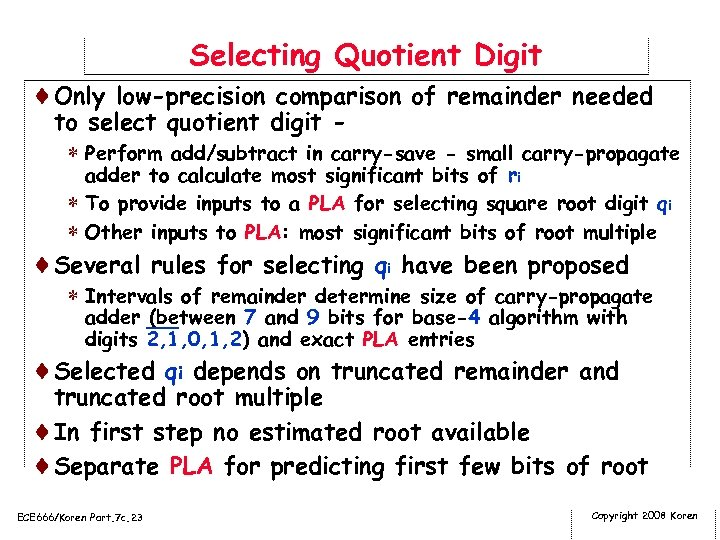Selecting Quotient Digit ¨Only low-precision comparison of remainder needed to select quotient digit * Perform add/subtract in carry-save - small carry-propagate adder to calculate most significant bits of ri * To provide inputs to a PLA for selecting square root digit qi * Other inputs to PLA: most significant bits of root multiple ¨Several rules for selecting qi have been proposed * Intervals of remainder determine size of carry-propagate adder (between 7 and 9 bits for base-4 algorithm with digits 2, 1, 0, 1, 2) and exact PLA entries ¨Selected qi depends on truncated remainder and truncated root multiple ¨In first step no estimated root available ¨Separate PLA for predicting first few bits of root ECE 666/Koren Part. 7 c. 23 Copyright 2008 Koren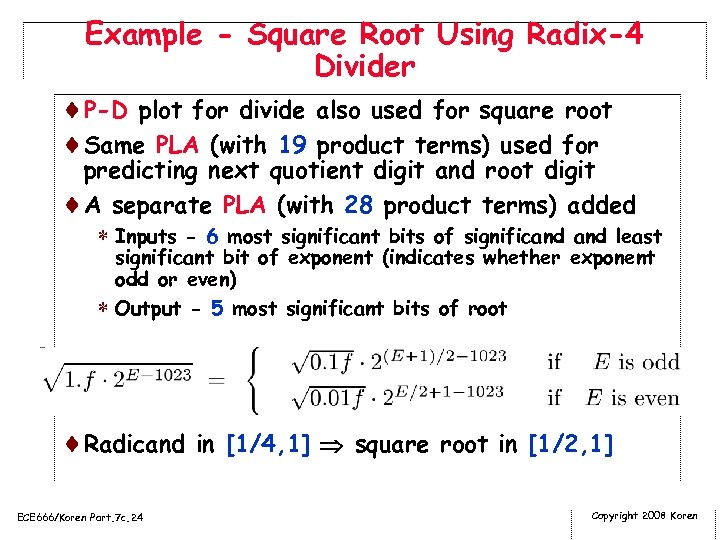Example - Square Root Using Radix-4 Divider ¨P-D plot for divide also used for square root ¨Same PLA (with 19 product terms) used for predicting next quotient digit and root digit ¨A separate PLA (with 28 product terms) added * Inputs - 6 most significant bits of significand least significant bit of exponent (indicates whether exponent odd or even) * Output - 5 most significant bits of root ¨Radicand in [1/4, 1] square root in [1/2, 1] ECE 666/Koren Part. 7 c. 24 Copyright 2008 Koren